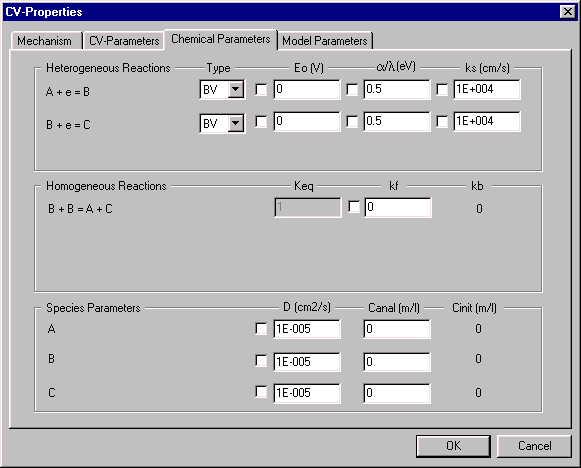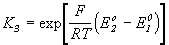# Digisim® Tutorials

#### 7. Thermodynamically Superfluous Reactions

A thermodynamically superfluous reaction (TSR) is a reaction whose presence or absence does not alter the chemical equilibria of any of the species in the system. One important property of such reactions is that the thermodynamic parameter for this reaction (Eo or Keq) is related to the thermodynamic parameters of other reactions in the system, and hence does not have to be independently entered by the user.

DigiSim® automatically recognizes TSRs. The correct value for this thermodynamic parameter is calculated from the thermodynamic parameters already entered, and is inserted into the appropriate data box. This operation is illustrated below for the following disproportionation mechanism:

 A + e = B (Eo = 0 V) B + e = C (Eo = 0 V) 2B = A +C

First, enter this mechanism, then open the Chemical Parameters dialog box. Note that the thermodynamic parameter can be entered for any of the three reactions. Enter Eo = 0 for the two electron transfer reactions, and a value of 1 is automatically entered for Keq when the input box is clicked.Figure 1. Chemical Parameters dialog box.

It should also be noted that the box to the left of this field has disappeared, since this value cannot be independently varied. Keq for this reaction is related to the two redox potentials by the following equation:In order to be able to vary the value of Keq independently, the value for one of the redox potentials must be deleted. For example, delete the value for the reaction B + e = C, and then enter a value of 10 for Keq for the reaction 2B = A + C. The reaction B + e = C is now the TSR, and a value of 0.059 is shown for Eo.

Some other mechanisms that have TSRs are shown below (see also the catalytic mechanism illustrated in Tutorial #5).

ECE

A + e = B
B = C
C + e = D
B + C = A + D

Square Scheme

A + e = B
B = C
D + e = C
A = D
A + C = B + D

It is important to note that a TSR is not kinetically superfluous, and can have a significant effect of the current response for a given mechanism. Therefore, the kinetics effects of TSRs should always be considered.

DigiSim is a registered trademark of Bioanalytical Systems, Inc.

Copyright 1995-2000, BASi® E'CHEM Electrochemical Products Group

Back to Tutorials Index